Polynomials are compex to solve. Sometimes they can be solved quite easily and sometimes it seems like solving them is impossible. Fortunately there exists something called Newton’s Method which lets you approximate roots of a polynomial by using the Taylor Series.

The Taylor Series is defined as followsBy using the first two terms of the Taylor Series, we can approximate a function at some differentiate point.You need to first find an approximation point to where a root could possibly be, for example by looking at the function’s graph or by iterating through some values of x and watching for close values to zero or changes of sign.

For example let’s take the function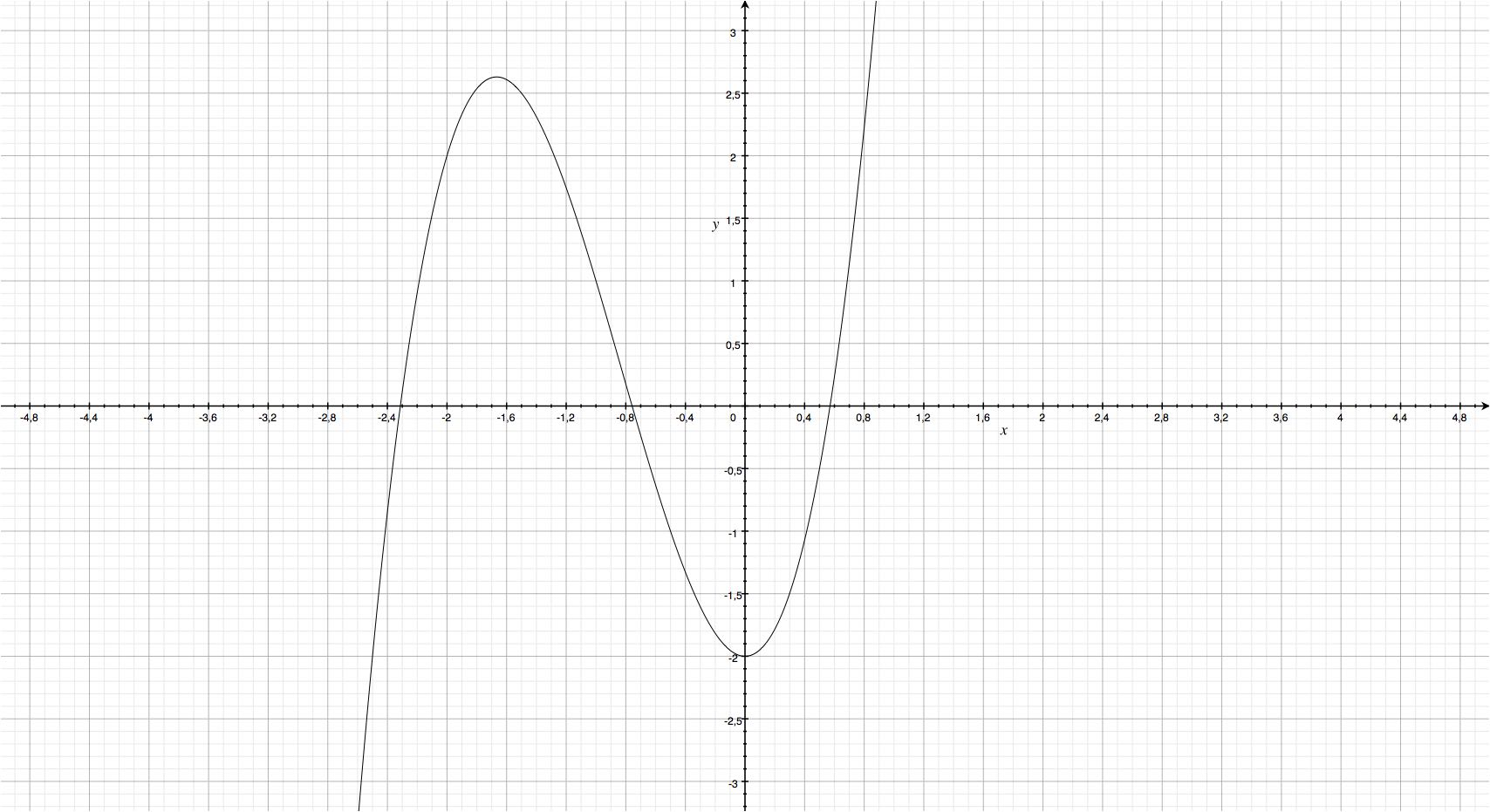You can see that there is some root between 0.5 and 0.75, we can take that as approximation.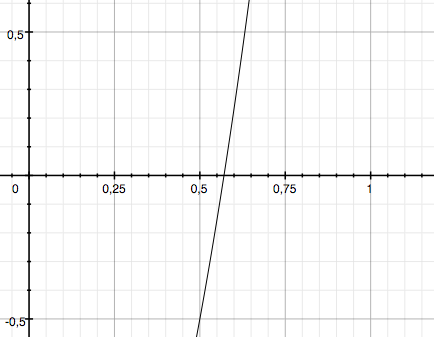Letbe our initial guess. Then, letbe our new function that will get assigned the approximated function that will be solved later.Then we find thatIf we plotyou can see that it is tangent to. Furthermore it has a root which is very close to the root we want to calculate. Dazzling!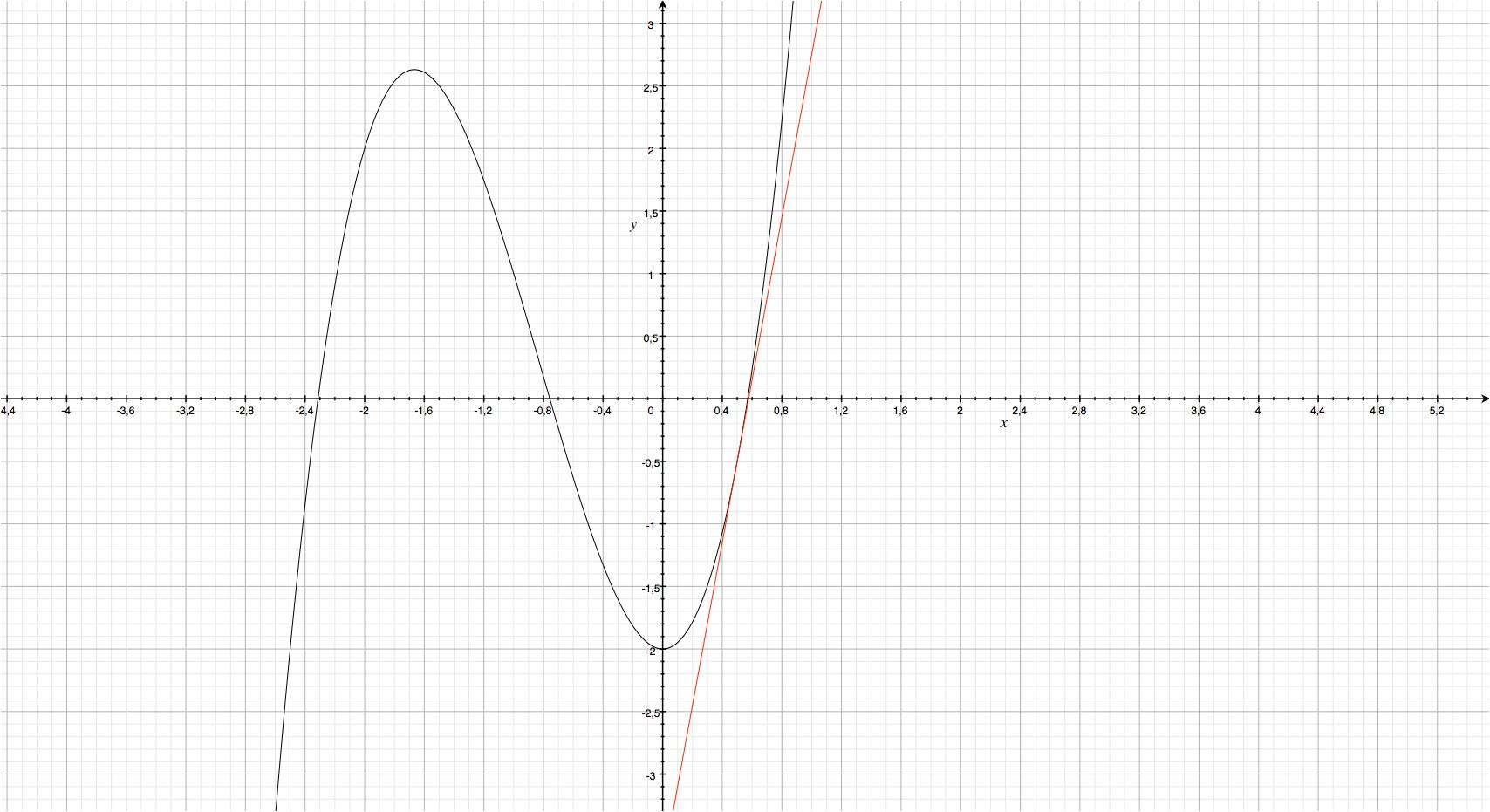Now, if we solvewe get. If we zoom in a little more, we find that the approximation turned out to be quite effective with our error of 0.06!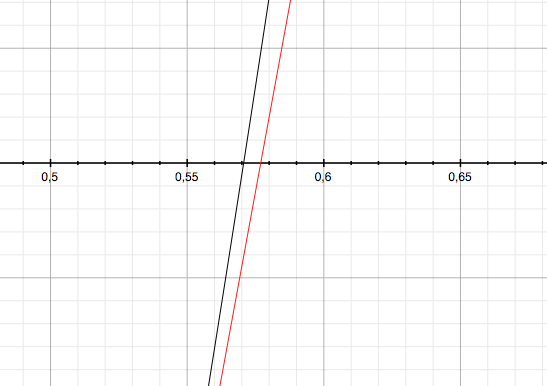If you repeat this process again with, you find that you get a new functionwhich has a root at. As seen, the method is quite effective and converges very quickly.

Iteration

Since we want to iterate this mathematical process with a computer, we might find it simpler if we simplify the equation. We can generalize the equation by noticing the pattern, that iswhen solved forbecomesWhich is in terms of programming much clearer to our eye than the mess above.

Example: Calculate the square root of a number

Getting the square root of some numberjust means solving the function. Since we find the derivative to bewe find that implementing such program to be pretty easy.

First, notice how quickly Newton’s Method converges for solving.

<td>
Starting at 2
</td>

<td>
Starting at 10
</td>

<td>
Starting at 100
</td>

<td>
1.5
</td>

<td>
5.1
</td>

<td>
50.1
</td>

<td>
1.4166666667
</td>

<td>
2.746078431
</td>

<td>
25.024996
</td>

<td>
1.4142135624
</td>

<td>
1.4442380949
</td>

<td>
12.55245805
</td>

<td>
1.4142135624
</td>

<td>
1.4145256551
</td>

<td>
6.355894
</td>

<td>
1.4142135624
</td>

<td>
1.4142135968
</td>

<td>
3.335281609
</td>

 Step 1 2 3 4 5

As you can see Newton’s Method converges pretty fast, even if your approximation is absurdly dumb. Such algorithm can be implemented with ease as seen below (C code).

#include <stdio.h>
#include <stdlib.h>
#include <math.h>

int main(int argc, char **argv)
{
double x, num;
int i;

if(argc != 2) {
printf("Usage: %s number\n", argv);
return 1;
}

num = atof(argv);

// First guess
x = num/2.0;

// Number of iterations
i = 25;

while(i--) {
x = -(pow(x, 2.0) - num)/(num * x) + x;
}

printf("%f\n", x);
return 0;
}

The program though turns out to have big errors for numbers bigger than 10. Notice how bigger the number the worse the approximation? This happens because our iteration count and approximation is only efficient for very small numbers.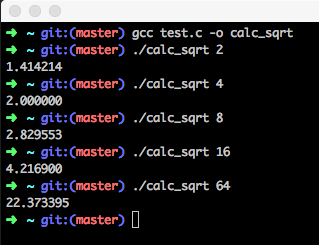There are a multitude of improvements that could be done such as making the iteration count depend on the number as well as trying to find a good linear approximation to the square root. I won’t discuss these methods here since my only ambition was to introduce you to Newton’s Method and give you an idea of how it works and how awesome it is.